# 2nd PUC Chemistry Question Bank Chapter 1 The Solid State

## Karnataka 2nd PUC Chemistry Question Bank Chapter 1 The Solid State

### 2nd PUC Chemistry The Solid State NCERT Textbook Questions and Answers

Question 1
Define the term ‘amorphous’. Give a few examples of amorphous solids.
Amorphous or amorphous solids imply those solids in which the constituent particles have short-range order. These have irregular shapes and are isotropic in nature. Apart from that, they do not have sharp melting points. A few examples of amorphous solids are glass, rubber, plastic, celluose etc.

Question 2.
What makes a glass different from a solid such as quartz? Under what conditions could quartz be converted into glass?
Quartz is a crystalline solid whereas glass is an amorphous solid. Quartz can be converted into glass by melting and rapid cooling.

Question 3.
Classify each of the following solids as ionic, metallic, molecular, network (covalent) or amorphous.
(i) Tetra phosphorus deioxide (P4O10)
(ii) Ammonium phosphate (NH4)3PO4
(iii) SiC
(iv) I2
(v) P4
(vi) Plastic
(vii) Graphite
(viii) Brass
(ix) Rb
(x) LiBr
(xi) Si
Amorphous solids: Polyurethane, cellophane, polyvinylchloride, fiberglass.
Crystalline solids: Naphthalene, benzoic acid, Teflon, potassium nitrate, copper.Question 4.
(i) What is meant by the term ‘coordination number’?
(ii) What is the coordination number of atoms:
(a) in a cubic close-packed structure?
(b) in a body-centered cubic structure?
(a) The co-ordination number of a constituent particle (atom, ion, or molecule) is the number of nearest neighbours in its contact in the crystal lattice.
(b) (i) Twelve,
(ii) Eight.

Question 5.
How can you determine the atomic mass of an unknown metal if you know its density and the dimension of its unit cell? Explain.
The atomic mass can be determined using the formula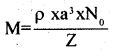where ρ is the density of the metal, a is the dimension of the unit cell, N0 is the Avogadro number and Z is the number of atoms in the unit cell.

Question 6.
(a) ‘Stability of a crystal is reflected in the magnitude of its melting points’.
(b) The melting points of some compounds are given below:
Water = 273K, ethyl alcohol = 155.7 K, Diethyl ether = 156.8K, Methane = 90.5K.
What can you say about intermolecular forces between these molecules?
(a) The stability of a crystal depends upon the magnitude of the force of interaction in the constituting particles. Greater the force of attraction present more will be the stability of the crystal. For example, ionic solids such as Naci, KCl, etc. have very high melting and boiling points while the molecular crystals such as naphthalene, iodine, etc. have low values of melting and boiling points.

(b) The melting points of different substances are:

• Ice = 273 K
• Ethyl alcohol = 155.7 K
• Diethyl ether = 156.8 K
• Methane = 90-5 K

The intermolecular forces in molecules of ice and ethyl alcohol are mainly hydrogen bonding. The magnitude is more in ice than in alcohol as is evident from the values of melting points.

The forces in the molecules of diethyl ether are dipolar forces while in methane, these are mainly the van der Waal’s forces which is quite evident from its melting point (least among the compounds listed).

Question 7.
How will you distinguish between the following pairs of terms:
(i) Hexagonal close-packing and cubic close-packing?
(ii) Crystal lattice and unit cell?
(iii) Tetrahedral void and octahedral void?
(i) When a third layer is placed over the second layer in such a way that the spheres cover the tetrahedral or ‘C’ voids, a three dimensional closest packing is obtained where the spheres in every third or alternate layers are vertically aligned (i.e. the third layer is directly above the first, the fourth above the second layer or so on) calling the first layer as layer A and the second layer as layer B, the arrangement is ABAB pattern of hexagonal close packing.

When the third layer is placed over the second layer in such a way that spheres cover the octahedral or ‘d’ voids, a layer different from layers A and B is produced. Let us call it layer C, continuing further, a packing is obtained where the spheres in every fourth layer will be vertically aligned. This pattern of stocking spheres is called ABCABC… pattern or close cube packing (CCP).

(ii) A regular three-dimensional arrangement of points in space is called a crystal lattice.
The unit cell is the smallest portion of a crystal lattice which, when repeated in different directions, generates the entire lattice.

(iii) Wherever a sphere of the second layer is above the void of the first layer (or vice versa) a tetrahedral void is formed. These voids are called tetrahedral voids because a tetrahedron is formed when the center of these four spheres is joined.
The voids which are surrounded by six spheres are called octahedral voids.Question 8.
How many lattice points are there in one unit cell of each of the following lattices?
(i) Face-centred cubic
(ii) Face-centred tetragonal
(iii) Body-centred
(a) In the face-centered cubic arrangement,
Lattice points located at the corners of the cube = 8
Lattice points located at the center of each face = 6
Total no. of lattice points = 8 + 6 = 14

(b) In face-centered tetragonal, the number of lattice points is also the same i.e., 8 + 6 = 14.
(In both the cases, particles per unit cell = 8 xX 1/8 + 6 x 1/2 = 4)

(c) In the body-centered cubic arrangement,
Lattice points located at the corners of the cube = 8
Lattice points located in the centre of the body = 1
Total no. of lattice points = 8 + 1 = 9
(the total number of particles per unit cell = 8 x 1/8 + 1 = 2)

Question 9.
Explain:
(i) The basis of similarities and differences between metallic and ionic crystals.
(ii) Ionic solids are hard and brittle.
(a) Similarities (i) Both ionic and metallic crystals have electrostatic forces of attraction. In ionic crystals, these are between the oppositely charged ions. In metals, these are among valence electrons and the kernels. That is why both have a high melting point.

(ii) in both cases the bond is non-directional Differences: (i) In ionic crystals, the ions are not free to move. Hence, they cannot conduct electricity in a solid-state. They can do so only in the matter state or in aqueous solutions. In metals, the electrons are free to flow. Hence they can conduct electricity in a solid-state.

(ii) Ionic bond is strong due to electrostatic forces of attraction.
Metallic bonds may be weak or strong depending on the number of valance electrons and size of the kernels.

Question 10.
Calculate the efficiency of packing in the case of a metal crystal for
(i) simple cubic
(ii) body-centered cubic
(iii) face-centered-cubic
(with the assumptions that atoms are touching each other).
(i)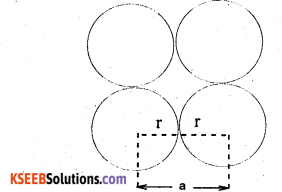Top/Bottom/side view of simple cubic unit cell As spheres are touching each other, evidently a = 2 r
Number of spheres per unit cell =$$\frac { 1 }{ 2 }$$ × 8 = 1
volume of the sphere = $$\frac { 4 }{ 3 }$$πr3
volume of the cube = a3 = (2r)3 = 8r3
∴ Fraction occupied, i.e. packing fraction
($$\frac { 4 }{ 3 }$$πr3) / 8r3
or % occupied = 52.4%.(ii)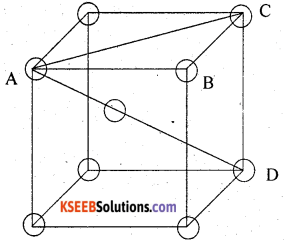spheres at the corners, body diagonal, AD = 4r further, face diagonal,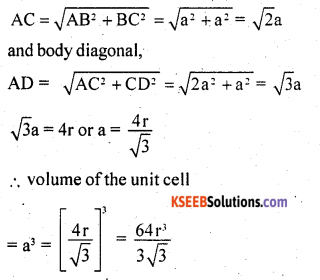Number of spheres per unit cell = 8 × $$\frac { 1 }{ 8 }$$ + 1 = 2
volume of 2 spheres = 2 × $$\frac { 4 }{ 3 }$$ πr3 = $$\frac { 8 }{ 3 }$$ πr3
∴ Fraction occupied i.e. packing fraction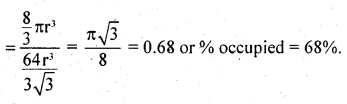(iii)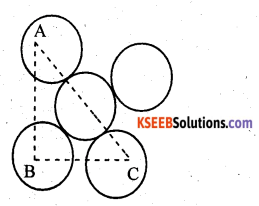A, B, C are the centers of the sphere. A sphere on the face centre is touching the spheres at the corners evidently AC = 4r
But from the right-angled triangle ABC∴ volume of the unit cellNumber of spheres in the unit cell
= 8 × $$\frac { 1 }{ 8 }$$ + 6 × $$\frac { 1 }{ 2 }$$ = 4
volume of 4 spheres = = 4 × $$\frac { 4 }{ 3 }$$ πr3 = $$\frac { 16 }{ 3 }$$ πr3
∴ fraction occupied, i.e. packing fraction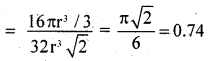or % occupied = 74%.

Question 11.
Silver crystallizes in fee lattice. If the edge length of the cell is 4.07 x 10-8 cm and density is 10.5 g cm-3, calculate the atomic mass of silver.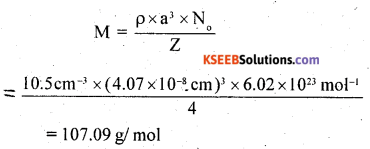Question 12.
A cubic solid ¡s made of two elements P and Q. Atoms of Q are at the corners of the cube and P at the body centre. What is the formula of the compound? What are the coordination numbers of P and Q?
As atoms, Q are present at the eight comers of the cube, therefore, the contribution of atoms of Q in the unit cell = 1/8 x 8 = 1.
As atom P is present at the body centre, therefore, the contribution of atoms of P in the unit cell = 1.
Ratio of atoms of P: Q = 1:1
Hence, the formula of the compound = PQ The coordination number of each P and Q = 8.

Question 13.
Niobium crystallizes in a body-centered cubic structure. If the density is 8.55 g cm-3, calculate the atomic radius of niobium using its atomic mass 93 u.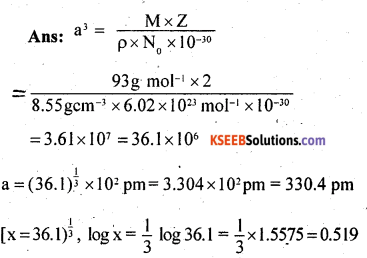or x = antilog0.519 = 3.304 for body – centred cubic,
r = $$\frac{\sqrt{3}}{4}$$ a = 0.433a = 0.433 x 330.4 pm = 143.1 pm

Question 14.
If the radius of the octahedral void is r and the radius of the atoms in close packing is R, derive a relation between r and R.A sphere fitting into the octahedral void is shown by a shaded circle. A sphere above and a sphere below this small (shaded) sphere have not been shown in the figure. Obviously, ABC is a right-angled triangle. Applying Pythagoras theorem,
BC2 = AB2 + BC2Question 15.
Copper crystallizes into a fee lattice with an edge length of 3.61 x 10-8 cm. Show that the calculated density is in agreement with its measured value of 8.92 g cm-3.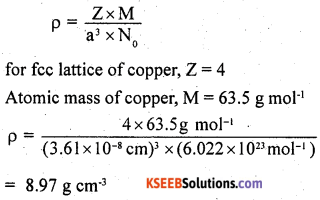which is in close agreement with the measured value.

Question 16.
The analysis shows that nickel oxide has the formula Ni098 O1.00. What fractions of nickel exist as Ni2+ and Ni3+ ions?
Let there are x ions of Ni2+ and (0.98 – x) ions of Ni3+.
For electrical neutrality of the compound :
Total positive charge contributed by Ni2+ and Ni3+ ions = Total negative charge contributed by
O2- ions
(+2 × x) + {+3 × (0-98 – x)} = 2
2x + 2.94 – 3x = 2
x = 0.94.
Thus, fraction of Ni2+ = $$\frac { 0.94 }{ 0.98 }$$ = 0.96 or 96%
Fraction of Ni3+ = (1 – 0.96) = 0.04 or 4%.

Question 17.
What is a semiconductor? Describe the two main types of semiconductors and contrast their conduction mechanism.
Substances whose conductance lies in between that of metals (conductors) and insulators are called semiconductors. Two main types of semiconductors are n-type and p-type. n-type semiconductor: When a silicon crystal is doped with atoms of group-15 elements, such as P, As, Sb or Bi, then only four of the five valance electrons of each impurity atom participate in forming covalent bonds and the fifth electron is almost free to conduct electricity. Silicon that has been doped with group-15 element is called n-type semiconductor.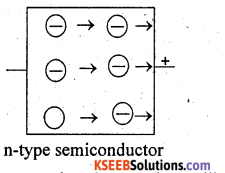(ii) p-type semiconductor: when a silicon crystal is doped with atoms of group-13 elements, such as B, Al, Ga, or In, each impurity atom forms only three covalent bonds with the host atoms. The place where the fourth electron is missing t is called a hole which moves through the crystal-like positive charge and hence increases its conductivity. Silicon that has been doped with group-13 element is a p-type semiconductor.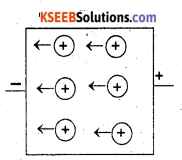Question 18.
Non-stoichiometric cuprous oxide, Cu2O can be prepared in the laboratory. In this oxide, the copper to oxygen ratio is slightly less than 2:1. Can you account for the fact that this substance is a p-type semiconductor?
The ratio less than 2:1 in Cu2O shows that some cuprous (Cu2+) ions have been replaced by cupric (Cu2+) ions. In order to maintain the electrical neutrality, every two Cut ions will be replaced by one Cu2+ ion which results in creating cation vacancies leading to positive holes. Since the conduction is due to positive holes, it is a p-type semiconductor.

Question 19.
Ferric oxide crystallizes in a hexagonal close-packed array of oxide ions with two out of every three octahedral holes occupied by ferric ions. Derive the formula of the ferric oxide.
O2- ions form the cubic close-packed lattice
Number of O2- = 8 x $$\frac { 1 }{ 8 }$$ = 1
Number of Fe3+ = $$\frac { 2 }{ 3 }$$ octahedral voids = $$\frac { 2 }{ 3 }$$
Formula of the compound =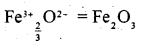Question 20.
Classify each of the following as being either a p-type or an n-type semiconductor: (i) Ge doped with In (ii) B doped with Si.
(a) p-type semi conductor
(b) n-type semi conductor.
(i) Ge is group 14 element and In is group 13 element. Hence, an electron-deficient hole is created, and therefore, it is a p-type semiconductor.
(ii) B is group 13 element and Si is group 14 element, there will be a free electron, So, it is an n-type semiconductor.

Question 21.
Gold (atomic radius = 0.144 nm) crystallizes in a face-centered unit cell. What is the length of a side of the cell?
Given r = 0.144 nm, a = ?
For fcc, a = 2$$\sqrt { 2 }$$ r = 2 × 1.414 × 0.144 nm = 0.407 nm

Question 22.
In terms of band theory, what is the difference
(i) between a conductor and an insulator
(ii) between a conductor and a semi¬conductor?
(i) The energy gap between the valence band and conduction band in an insulator is very large whereas in a conductor, the energy gap is very small or there is overlapping between the valance band and conduction band.
(ii) In a conductor, there is a very small energy gap or there is overlapping between the valence band and conduction band but in a semiconductor, there is always a small energy gap between them. ’

Question 23.
Explain the following terms with suitable examples:
(i) Schottky defect
(ii) Frenkel defect
(iii) Interstitials and
(iv) F-centres.
(i) Schottky defect:
It arises due to the missing of equal number of cations and anions from their normal positions leaving behind a pair of holes. This defect is usually observed in ionic compounds having high coordination number and ions of almost similar size. The density of the crystal is lowered due to the presence of vacancies in the crystal lattice. Schottky defect increases slightly the electrical conductance of the crystal. E.g. NaCl, KC1, CsCl.

(ii) Frenkel defect:
It arises w’hen an ion, usually cation, leaves its normal site and occupies an interstitial site. This defect is usually observed in ionic ‘ compounds having low co-ordination number , and crystals with anions are much larger in size than the cations. Since no ions are missing from the crystals as whole, it doesn’t affect the density of the crystal.
E.g.: AgCl, ZnS, AgBr

(iii) Interstitials:
Atoms or ions which occupy the normally vacant interstitial positions in a crystal are called interstitials.

(iv) F-centres:
The electron occupying holes, created by the missing anions from the lattice sites are called F-centres. These F-centres are responsible for colour of the compound.

Question 24.
Aluminum crystallises in a cubic close-packed structure. Its metallic radius is 125 pm.
(i) What is the length of the side of the unit cell?
(ii) How many unit cells are there in 1.00 cm3 of aluminum?
(i) For CCP structure, edge length, a = 2√2r = 2√2 x 125pm = 354pm
(ii) volume of unit cell = a3 = (354 × 10-8m)3
∴ Number of unit cells in 1.00 cm3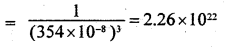Question 25.
If NaCl is doped with 10-3 mol% of SrCl2, what is the concentration of cation vacancies?
Introduction of one Sr2+ introduces one cation vacancy because Sr2+ replaces two Na+ ions. Introduction of 10-3 moles of SrCl2 per 100 moles of NaCl would introduce 10’3 mole cation vacancies
∴ Number of vacancies per mole of NaCl
= $$\frac{10^{-3}}{100}$$ = 10-5 mole = 10-5 x 6.02 x 1023 1 (F = 6.02 x 1018 vacancies.

Question 26.
Explain the following with suitable examples:
(i) Ferromagnetism
(ii) Paramagnetism
(iii) Ferrimagnetism
(iv) Antiferromagnetism
(v) 12 – 16 and 13 – 15 group compounds.
(i) Ferromagnetism is considered as an extreme case of paramagnetism. Those substances with a strong attraction to an external magnetic field and those retain a permanent magnetism even when the field is removed are known as ferromagnetic substances. Ferrimagnetism is caused by the spontaneous alignment of the magnetic moment of atoms or ions in the same direction. Examples of ferromagnetic substances are Fe, Co, Ni, CrO2 alloys of Fe, Co and Ni, etc. Once such material is, magnetised, it remains permanently magnetized Ferromagnetic(ii) Paramagnetic substances are those which are attracted by magnetic field and have impaired electrons. They lose magnetism in the absence of magnetic field. The greater the number of unpaired electrons, the greater the magnetic moment of the substance and hence greater the paramagnetism. E.g.: O2, CuO, Fe3+, Al, Mn, Cu2+ etc.

(iii) These are substances which are expected to have large magnetism on the basis of unpaired electrons but actually have small net magnetic moment. This is due to the alignment of magnetic moment in opposite direction in unequal numbers resulting in a net magnetic moment.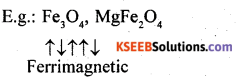(iv) These are substances which are expected to possess paramagnetism or ferromagnetism on the basis of unpaired electrons but they actually possess zero net magnetic moment. It arises due to the alignment of magnetic moments in opposite direction in a compensatory manner resulting a zero magnetic moment.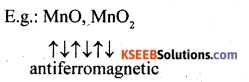(v) Germanium and silicon are group 14 elements and have a characteristic valance of four and form four bonds as in diamond. A large variety of solid-state materials have been prepared by a combination of groups 13 and 15 or 12 and 16 to stimulate average valence of four as Ge or Si. Typical compounds of group 13 -15 are InSb, AlP, and GaAs. Gallium arsenide (GaAs) semiconductors have very fast response and have revolutionized semiconductor devices. ZnS, CdS, CdSe, and HgTe are examples of group 12 – 16 compounds. In. these compounds, the bonds are not perfectly covalent and the ionic character depends on the electronegativities of the two elements.

### 2nd PUC Chemistry The Solid State One Mark Questions and Answers

Question 1.
How many atoms are present in the unit
cell of
(a) simple cubic lattice
(b) BCC
(c) FCC
(a) 1
(b) 2 and
(c) 4.

Question 2.
How can you convert NaCl structure into CsCl structure and vice versa?
NaCl structure can be converted into CsCl. structure by application of pressure while the reverse can be done by heating to 760 k.

Question 3.
How does amorphous silica differ from quartz?
In amorphous Silica, SiO4 tetrahedra are randomly joined to each other whereas in quartz, they are linked in a regular manner.

Question 4.
What are stoichiometric defects or intrinsic defects in ionic crystals?
Stoichiometric defects are those defects in which the ratio of cations to anions remains the same are represented by the molecular formulaQuestion 5.
What is the effect of the presence of Schottky defects on the density of a crystal?
The density of the crystal decreases.

Question 6.
Name one Solid which has both Schottky and Frenkel defects. !
Silver bromide (AgBr).

Question 7.
What is Frenkel, defect?
When some ions (usual cations) are missing from the lattice sites and they occupy the interstitial sites so that electrical neutrality, as well as stoichiometric ratio, is maintained, it is called Frenkel defect.

Question 8.
Why does the conductivity of metals decrease with the rising temperature?
The kernels begin to vibrate in the path of the flow of electrons creating hindrance in the flow.

Question 9.
What is the type of lattice imperfections found in crystals?
(a) Stoichiometric defects, viz, Schottky defect and Frenkel defect and
(b) Non-Stoichiometric defects, viz., metal excess, metal deficiency and
(c) Impurity defects.

Question 10.
Define the coordination number of a metal ion in an ionic crystal.
In an ionic crystal, the coordination number of a metal ion (+ve ion) is the number of negative ions surrounding the metal ion, i.e. which are present as its nearest neighbours.

Question 11.
Why are amorphous solids to be considered supercooled liquids?

• Like liquids, amorphous solids are isotropic
• Like liquids, they possess fluidity.### 2nd PUC Chemistry The Solid State Two Marks Questions and Answers

Question 1.
The Refractive index of a solid is observed to have the same value along with all directions. Comment on the nature of solid. Would it show cleavage property?
As the solid has the same value of refractive index along with all directions. This means that it is isotropic and hence amorphous. Being an amorphous solid, it would not show a clean cleavage when cut with a knife. Instead, it would break into pieces with irregular surfaces.

Question 2.
Ionic solids conduct electricity in a molten state but not in a solid-state. Explain.
In a molten state, ionic solids dissociate to give free ions and hence can conduct electricity. However, in the solid-state, as the ions are not free but remain held together by strong electrostatic forces of attraction, they cannot conduct electricity in the solid-state.

Question 3.
Give reason.

1. When ZnO is heated, its electrical conductivity is increased.
2. NaCl is harder than Na.
3. Crystals of FeO usually show metal deficiency defects.

1. When ZnO is heated at a high temperature, it loses oxygen reversibly. The excess metal ions, (Zn2+) are accommodated in the interstitial positions and the electrons are trapped in the neighbourhood interstitial positions. These electrons enhance the electrical conductivity of ZnO.

2. NaCl is an ionic compound having a strong electrostatic force of attraction while Na has a metallic bond which is much weaker.

3. Fe can show variable oxidation states. Two out of three Fe3+ ions may be converted into Fe3+, and the third Fe2+ ion may leave from the crystal lattice. As a result, a metal deficiency defect is formed.

Question 4.
What type of defect can arise when a solid is heated? Which physical property is affected and in what way?